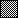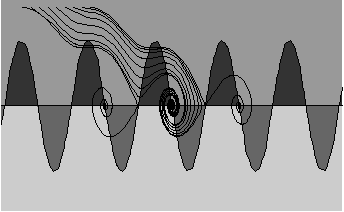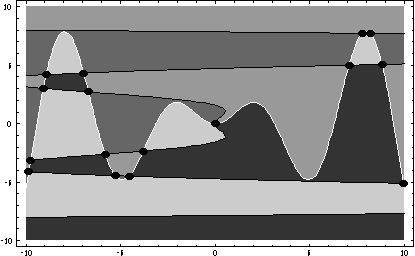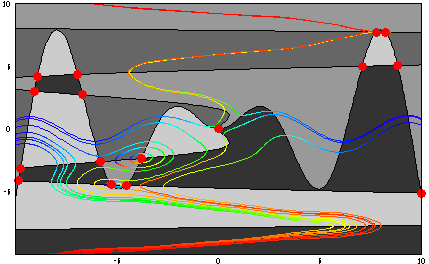##2. Coloring the Compass Directions

Given an autonomous system as in section 1, one can look at the individual curves f = 0 or g = 0. These are called nullclines and we have already seen they are quite easy to get using a contour plotting routine. It makes more sense to superimpose the two nullclines rather than attempting a contour plot of the product f*g. The following code produces a nullcline plot, though we omit the image since it is essentially identical to the last diagram in section 1.

In:=

If your output will be a sequence of graphs, select a display option.
Display as:

The intersection points correspond to equilibria, and we now know how to find them efficiently. But what of all the different regions? A moment's thought will convince you that within each region the compass direction of the underlying vector field is one of NE, NW, SE, or SW. Thus these regions can be a valuable tool in understanding and illustrating the orbit behavior.

Our task, then, is to somehow capture these regions as polygon objects and shade them using four shades of gray, with a different shade for each of the directions. One fairly straightforward approach comes to mind: simply generate a shaded contour plot (or a density plot) of 3 sign f + sign g; this sum takes on the nine values -4, -3, -2, -1, 0, 1, 2, 3, and 4, and so the shaded regions will be just what we want (assuming 0 does not occur as a sign, the range will be {-4, -2, 2, 4}. This works in theory, but it has two drawbacks for the serious investigator. First, it takes too long to get a decently resolved image, and second, there are often resolution problems near the borders: the gray regions tend to become jagged at the edges. Of course, the big advantage of this method is that the programming is totally trivial: just generate a single contour plot or density plot.

Dan Schwalbe and I came up with a totally different approach. It sounds complicated, and indeed it was very difficult to get right, but we now believe that the algorithm and its implementation correctly deal with the diverse cases that can arise. The advantages are that it is quite fast and produces highly accurate images. Before describing the algorithm, let us present an image so that the reader can grasp the message we are trying to convey.

Here is an image of the phase plane for a pendulum with friction, showing the shaded nullclines and several orbits, all but two of which converge to the central equilibrium. We have here converted the second-order equation x'' = -x' - 10 sin x to a first-order system. The function NullclinePlot, which accompanies this article, must be loaded.

In:=

If your output will be a sequence of graphs, select a display option.
Display as:

Out

Certainly the image becomes much more informative when we superimpose some orbits. We show how to do that using NDSolve (the full VisualDSolve package allows a one-line treatment of such combined images).

In:=

If your output will be a sequence of graphs, select a display option.
Display as:From now on we will generally suppress the Mathematica code. The preceding example should provide enough information for the reader to generate these as will as his or her own examples.
The next image shows the gray regions for our main example, followed by an image of the orbits. The shaded regions allow for a quicker and better interpretation of the orbit structure. The NullclineStyle option is used to distinguish the x-nullclines (black) from the y-nullclines (white).

In:=

If your output will be a sequence of graphs, select a display option.
Display as:Here is a view of some orbits, colored to represent increasing t (from red to blue).And here is an image of the CODEE teddy bears (cover of the Spring 1995 CODEE newsletter; also [BCB, p. 304]). This example shows that the algorithm is capable of dealing with a very complicated contour plot. This takes a bit of time (and memory) even on a fast computer! We are rewarded with a detailed view of the bear's phase plane and an unexpected glimpse of a latent bear: the contours at the bottom of the image form a bear's head!

In:=

If your output will be a sequence of graphs, select a display option.
Display as:

Out

Getting the shaded regions was not easy. Here is a bare outline of our algorithm. The main idea is to take the all the curves in the nullcline data produced by the contour plots and subdivide them at certain "critical" x-values. An x-value is critical if it is

* one of the x-bounds of the rectangle;

* the x-coordinate of a point at which a curve stops going left and starts going right, or vice
versa (i.e., a division point that refines the curve data into graphs of functions);

* the x-coordinate of an equilibrium point;

* the x-coordinate of a point where a contour leaves the rectangle at the top or the bottom;

* the x-coordinate of the first or last point in one of the contour data segments (e.g., a contour that is a closed curve has to start somewhere; that starting x-value will be critical).

Once all the contour data is partitioned so that each curve occupies a strip between consecutive critical x-values -- thus defining a function of x and not intersecting any other curve -- we are in good shape. For each piece of curve we can form the polygon whose bottom border is the curve and whose left and right sides run vertically all the way to the top. The idea is then to color these polygons appropriately; if they are sorted properly then, when one overlays another, its gray will paint over the gray of the one underneath. To sort, and to determine which shade of gray to use, we go to the center of the x-domain (the average of two adjacent critical x-values), and look at where the vertical line at this point intersects all the curves; we use this information to do our sorting, and we take the average of successive intersection points to get interior points at which to evalute f and g and so determine a gray shade. Whew! This is horrendously complicated! But it works well and because the data sets are not too large, it runs fairly quickly.

Note that the final images are a bit of an optical illusion. The first example, the pendulum, looks like 14 shaded polygons. But in reality there are 36 polygons, because the many strips formed by the critical x-values mean that the large polygons are made up of several smaller ones. The bear example, which visually appears as a gorup of about 100 polygons, actually consists of 921 smaller polygons.

Exercise. If the user wishes to shade in the regions where a field points N, S, E, and W (instead of NW, SW, NE, SE), that is possible via NullclinePlot[{f - g, f + g}, ***]. The equilibrium points will be visible in the same way.

There are several options to NullclinePlot (defaults in parentheses):

Nullclines sets whether the curves forming the nullclines are shown (True).

NullclineContourPlotPoints controls the resolutiuon of the contour
plots that get everything started (20).

ShowEquilibria causes a point to appear at each equilibrium point (False); its color is
controlled by EquilibriumPointColor (RGBColor[0, 0, 0]).

NullclineShadingMethod causes the underlying algorithm to switch to the density plot
method alluded to earlier. This is useful for the occasional example for which the
primary method fails (see the example based on the Dirac delta function in section 3).

NullclineStyle allows the user to set distinct styles for the x- and y-nullclines ({{}, {}}).